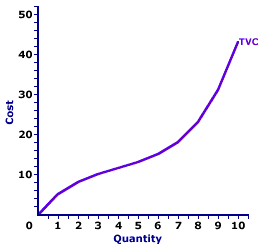Tuesday  October 3, 2023
 AmosWEB means Economics with a Touch of Whimsy!LONG-RUN AGGREGATE SUPPLY: The total (or aggregate) real production of final goods and services available in the domestic economy at a range of price levels, during a period of time in which all prices, especially wages, are flexible, and have achieved their equilibrium levels. Long-run aggregate supply (LRAS) is one of two aggregate supply alternatives, distinguished by the degree of price flexibility; the other is short-run aggregate supply (SRAS).TOTAL VARIABLE COST AND MARGINAL COST:

A mathematical connection between marginal cost and total variable cost stating that marginal cost IS the slope of the total variable cost curve. This relation between total variable cost and marginal cost is also seen with total cost. The slope of the total cost curve is marginal cost, as well. The relation between total variable cost and marginal cost is but another in the long line of applications of the total-marginal relation.
Total Variable Cost and Marginal CostThe slope of the total variable cost curve (and total cost curve) is marginal cost. As such, if the total variable cost curve has a positive slope (that is, is upward sloping), then marginal cost is positive. Moreover, if the total variable cost curve has a positive and increasingly steeper slope, then the marginal cost is positive and rising. If the total variable cost curve has a positive and increasingly flatter slope, then the marginal cost is positive but falling.

This particular total-marginal relation applies to both total variable cost and total cost. Because not only is marginal cost the slope of the total variable cost curve, it is also the slope of the total cost curve. The reason is that any changes in total cost resulting from changing output is matched by changes in total variable cost. This occurs because total fixed cost is FIXED.

This two-paneled graph for the production of Wacky Willy Stuffed Amigos (those cute and cuddly armadillos, turtles, and lizards) visually illustrates the connection between total variable cost and marginal cost. For the first few quantities of output (Stuffed Amigos), total variable cost in the top panel is positive AND the slope of the total variable cost curve decreases--it becomes flatter. This corresponds with a positive and decreasing marginal cost in the bottom panel up to 4 Stuffed Amigos. For the next several quantities of Stuffed Amigos output, the slope of the total variable cost curve becomes increasingly steeper. This corresponds to an increasing marginal cost in the bottom panel.

The prime conclusion is the key role played by the law of diminishing marginal returns in the slope of both the marginal cost curve and the total variable cost curve.

• The U-shape of the marginal cost curve is a direct reflection of first increasing marginal returns, as marginal cost falls to a minimum, then decreasing marginal returns and the onset of the law of diminishing marginal returns as marginal cost rises.

• However, because the marginal cost curve is a plot of the slope of the total variable cost curve, then the shape of the total variable cost curve also reflects the law of diminishing marginal returns. The flattening slope of the total variable cost curve for small quantities of output is due to increasing marginal returns. Then the onset of the law of diminishing marginal returns causes the total variable cost curve to become increasingly steeper.
While this diagram relates marginal cost and total variable cost, the same story applies to the relation between marginal cost and total cost. Marginal cost is also the slope of the total cost curve. As such, the shape of the total cost curve is also a reflection of increasing, then decreasing marginal returns.

 <= TOTAL VARIABLE COST TOTAL VARIABLE COST AND TOTAL PRODUCT =>Recommended Citation:

TOTAL VARIABLE COST AND MARGINAL COST, AmosWEB Encyclonomic WEB*pedia, http://www.AmosWEB.com, AmosWEB LLC, 2000-2023. [Accessed: October 3, 2023].

Check Out These Related Terms...

Or For A Little Background...

And For Further Study...
Search Again?WHITE GULLIBON[What's This?] Today, you are likely to spend a great deal of time touring the new suburban shopping complex looking to buy either a birthday greeting card for your grandmother or a coffee cup commemorating yesterday. Be on the lookout for door-to-door salesmen.Your Complete ScopeThe average length of a "business lunch" is about 36 minutes."Managing intellectual assets has become the single most important task of business. "-- Thomas Stewart, authorDDADemand Deposits AccountsA PEDestrian's Guide Xtra CreditTell us what you think about AmosWEB. Like what you see? Have suggestions for improvements? Let us know. Click the User Feedback link.| | | | | | | | | | |
| | | |

Thanks for visiting AmosWEB